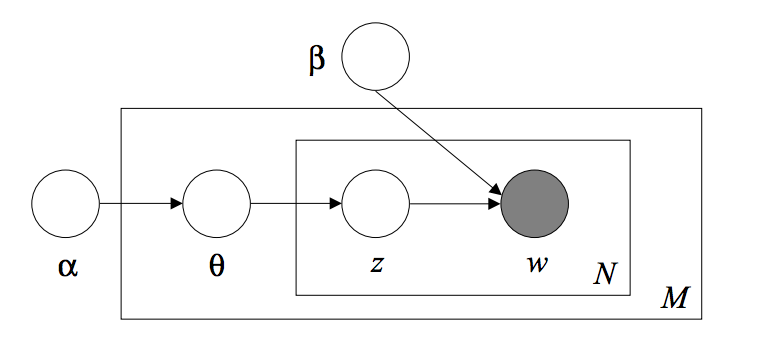LDA, the Most Commonly-Used Topic Model

I have been working on projects related to user profiles lately at Autodesk. An important part of the project are extracting information out of command usage data, of which Latent Dirichlet Allocation has been the main driving force. Hoping to get a better grasp of the underlying principles of LDA, I am reading this one of the most cited papers in Computer Science, by Blei, Ng and Jordan. Admittedly, the Bayesian math was a bit hard for me and I was not able to fully comprehend the material (never a big fan of Bayesian Statistics). Maybe I should have taken CS 228: Probabilistic Graphical Models

Intro

The goal of modeling text corpora is to find short descriptions of the text corpora while preserviing the essential statistical relationships. The basic methodology is to reduce each document in the corpus to a vector a real numbers. Important previous work includes TF-IDF, LSI and pLSI.

TF-IDF

• Counts are formed for number of occurences of each word and normalized to term frequency (TF)
• The inverse document frequency (IDF) measures the number of occurences of words in the entire corpus. - The end result is a term-by-document matrix whose columns contain the tf-idf values for each document in the corpus
• Shortcoming: relatively small amount of reduction in description length and reveals little in the way of intra-document statistical structure

Latent Semantic Indexing (LSI)

• Perform SVD on the X matrix to identify a linear subspace of tf-idf features that captures most of the variance
• Achieve significant compression in large collections

Probabilistic LSI (pLSI)

• Models each word in a document as a sample from a mixutre model where the mixture components are random variables that can be viewed as representations of topics
• Each Document is represented as a list of mixing proportions for these mixture components and thereby reduced to a probability distribution on a fixed set of topics
• Both LSI and pLSI relies on the fundamental assumption of exchangeability, which means that the order of words in a document can be neglected and the specific ordering of the documents in a corpus can also be neglected

Model

The basic idea of LDA is that documents are represented as random mixtures over latent topics, where each topic is characterized by a distribution over words. The LDA assumes follows the generative process of:

1. Decide the number of words in the document $N \sim \text{Poisson}(\zeta)$
2. Decide the topic mixture for the document $\theta \sim \text{Dir}(\alpha)$
3. For each of the $N$ words $w_n$:
• Choose a topic $z_n \sim \text{Multinomial}(\theta)$
• Choose a word $w_n$ from $p(w_n|z_n, \beta)$, a multinomial probability condiditioned on the topic $z_n$

The dimensionality $k$ of the Dirichlet distribution is the number of latent topics, which we assume is fixed and given. The $k$-dimensional Dirichlet random variable $\theta$ that parametrized the Multinomial Distribution that select the topics takes values in the $(k-1)$-simplex. The word probabilities $p(w_n|z_n, \beta)$ is parametrized by a $k \times V$ matrix $\beta$, which need to be estimated.

Given the parameters $\alpha$ an $\beta$, the joint distribution of a topic mixture $\theta$, a set of $N$ topics $z$ and a set of $N$ words $w$ is $$p(\theta, z, w|\alpha,\beta) = p(\theta|\alpha) \prod_{n=1}^N p(z_n|\theta) p(w_n|z_n,\beta)$$

Given that the distribution of topics $z$ sums up to 1 for a given set of parameter $\theta$, the marginal distribution of a topic mixture and a set of words is $$p(\theta, w|\alpha,\beta) = p(\theta|\alpha) \prod_{n=1}^N \sum_{z_n} p(z_n|\theta) p(w_n|z_n,\beta)$$

And the marginal distribution of a document can be obtained by integrating over $\theta$, $$p(w|\alpha,\beta) = \int p(\theta|\alpha) \left[ \prod_{n=1}^N \sum_{z_n} p(z_n|\theta) p(w_n|z_n,\beta) \right] d\theta$$

Finally, the product of the marginal probabilities of single documents is the probabilities of a corpus: $$p(D|\alpha,\beta) = \prod_{d=1}^M \int p(\theta_d|\alpha) \left[ \prod_{n=1}^{N_d} \sum_{z_{n,d}} p(z_{n,d}|\theta_d) p(w_{n,d}|z_{n,d},\beta) \right] d\theta_d$$

The LDA model is a probabilistic graphical model, as shown in the figure below. The parameters $\alpha$ and $\beta$ are corpus-level parameters and is sampled once in the process of generating the entire corpus. The variables $\theta_d$ are document-level variables and are sampled once per document. The variables $z_{n,d}$ and $w_{n,d}$ are word-level variables and are sampled once for each word in each document.Parameter Estimation

The parameter of the LDA model is estimated by maximizing the log likelihood of the corpus $$l(\alpha, \beta) = \sum_{d=1}^M \text{log} \ p(w_d|\alpha,\beta)$$ However, explicitly finding the maximum log likihood is often hard for models with latent random variables, and in this case the quantity $p(w_d|\alpha,\beta)$ cannot be computed tractably. In such setting, the expectation maximization (EM) algorithm comes to rescue. The strategy is to repeatedly construct a lower-bound on $l$ with methods invloved with Jensen’s inequality (E-step), and then optimize that lower-bound (M-step).

The LDA model in particular is solved by a variational EM algorithm, where the lower bound is constructed by the convexity-based variational inference

1. E-step: For each document, find the optimizing values of the variational parameters $\gamma_d^\ast, \phi_d^\ast$
2. M-step: Maximize the lower bound on the log likelihood with respect to the model parameters $\alpha$ and $\beta$, which corresponds to finding maximum likelihood estimates for each document under the approximate posterior computed in E-step